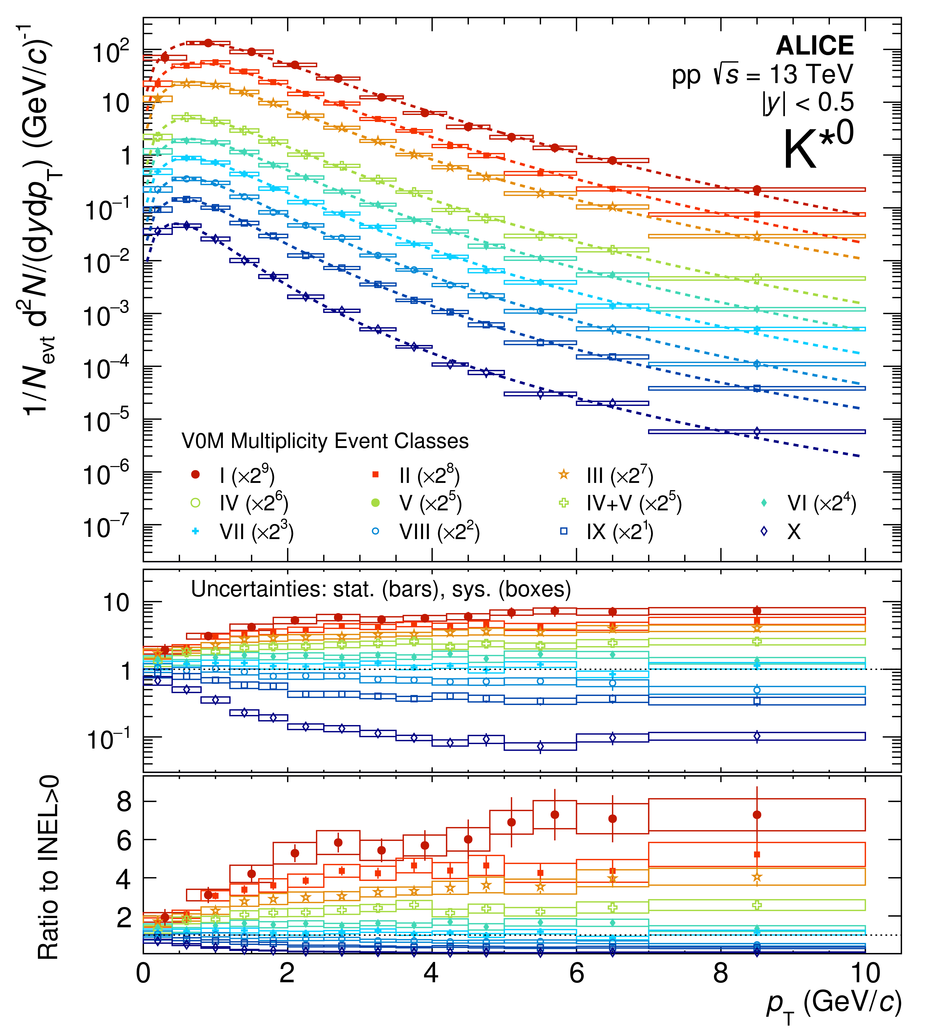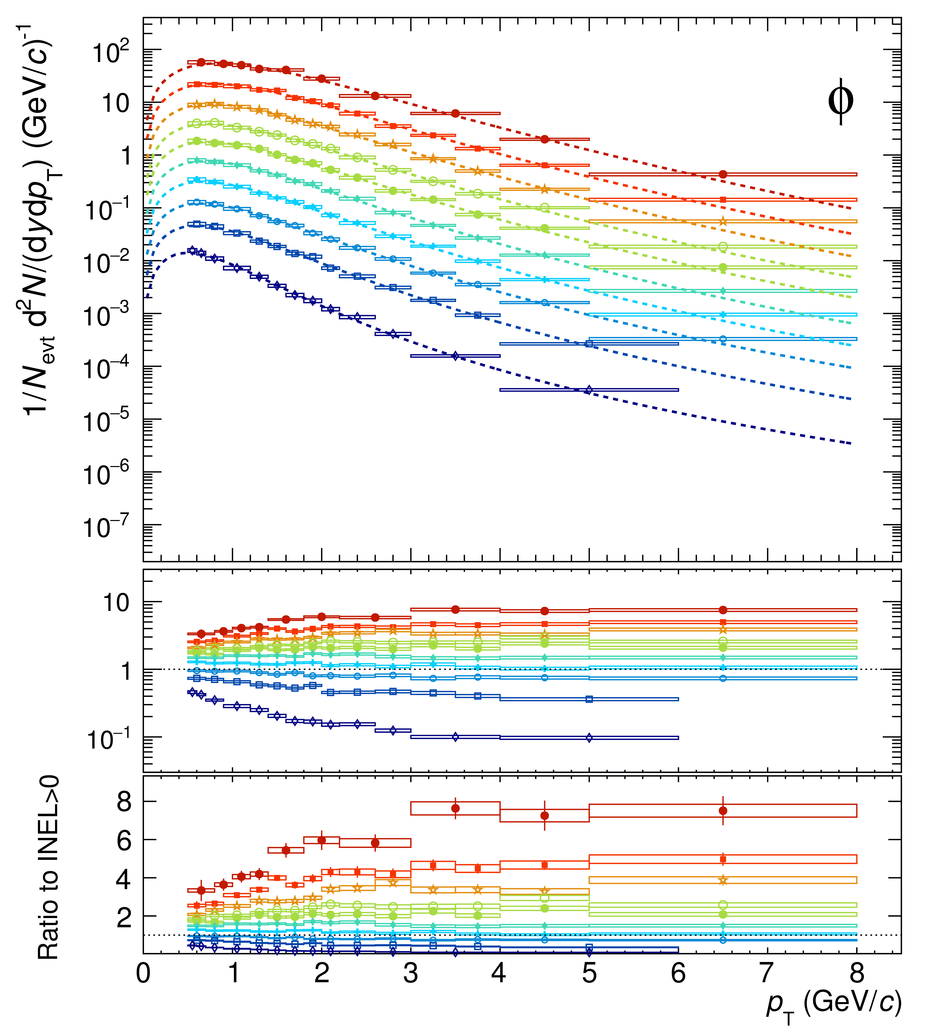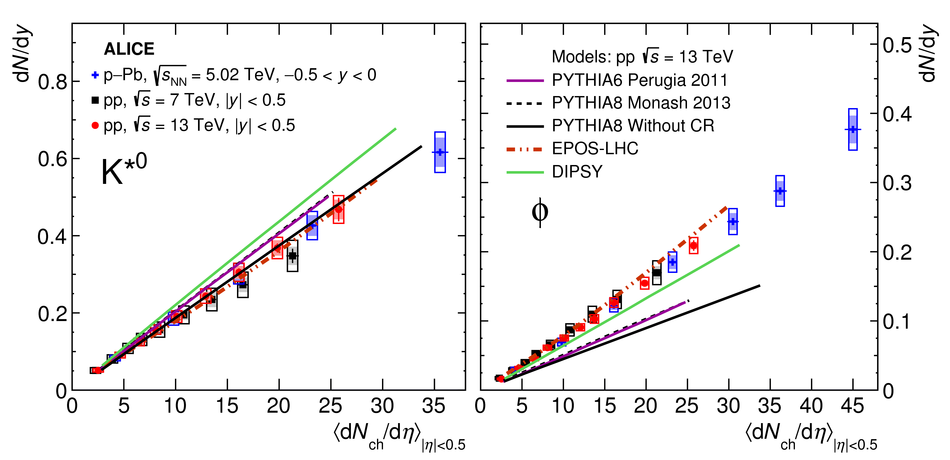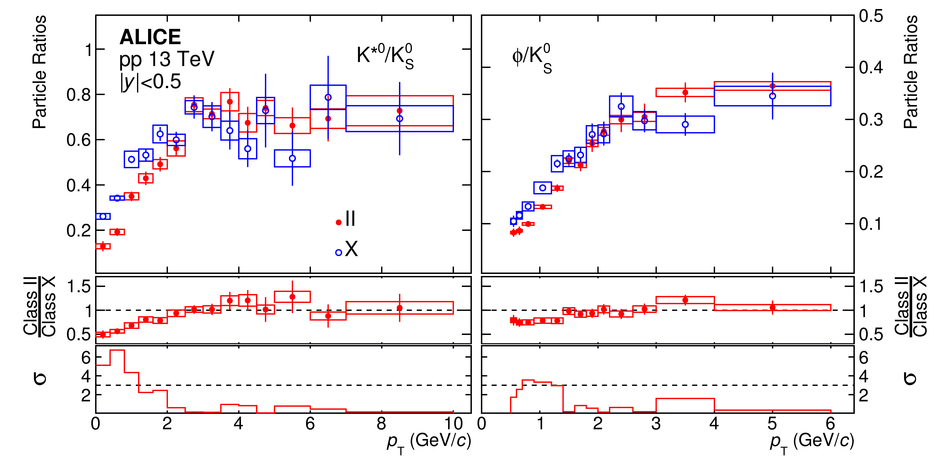# Multiplicity dependence of K*(892)$^{0}$ and $φ$(1020) production in pp collisions at $\sqrt{s}$ = 13 TeV

Measurements of identified hadrons as a function of the charged-particle multiplicity in pp collisions enable a search for the onset of collective effects in small collision systems. With such measurements, it is possible to study the mechanisms that determine the shapes of hadron transverse momentum ($p_{\rm{T}}$) spectra, to search for possible modifications of the yields of short-lived hadronic resonances due to scattering effects in the hadron-gas phase, and to investigate different explanations for the multiplicity evolution of strangeness production provided by phenomenological models. In this paper, these topics are addressed through measurements of the $\rm{K}^{*}(892)^{0}$ and $\phi(1020)$ mesons at midrapidity in pp collisions at $\sqrt{s}$ = 13 TeV as a function of the charged-particle multiplicity. The results include the $p_{\rm{T}}$ spectra, $p_{\rm{T}}$-integrated yields, mean transverse momenta, and the ratios of the yields of these resonances to those of longer-lived hadrons. Comparisons with results from other collision systems and energies, as well as predictions from phenomenological models, are also discussed.

Figures

## Figure 1

 \ptt spectra of \ks and \ph in pp collisions at \rs for different multiplicity classes, scaled by factors as indicated. The lower panels show the ratios of the multiplicity-dependent \ptt spectra to the multiplicity-integrated \igz spectra (with both linear and logarithmic vertical scales).## Figure 2

 Mean transverse momenta \mpt of \ks and \ph as functions of \dnc. Results are shown for pp collisions at \rs and 7~TeV~, as well as for \ppb collisions at \rsnn[5.02~TeV]~. The measurements in pp collisions at \rs are also compared to values from common event generators~. Bars represent statistical uncertainties, open boxes represent total systematic uncertainties, and shaded boxes show the systematic uncertainties that are uncorrelated between multiplicity classes (negligible for \ppb).## Figure 3

 Mean transverse momenta for \ks and \ph are compared with those for \kz, $\Lambda$+$\overline{\Lambda}$, $\Xi^{-}$+$\overline{\Xi}^{+}$, and $\Omega^{-}$+$\overline{\Omega}^{+}$ in pp collisions at \rs as a function of \dnc~. The values for $\Xi^{-}$+$\overline{\Xi}^{+}$ are shifted horizontally for visibility. Bars represent statistical uncertainties, open boxes represent total systematic uncertainties, and shaded boxes show the systematic uncertainties that are uncorrelated between multiplicity classes.## Figure 4

 \ptt-integrated yields \dndy of \ks (average of the particle and antiparticle) and \ph as functions of \dnc. Results are shown for pp collisions at \rs and 7~TeV~, as well as for \ppb collisions at \rsnn[5.02~TeV]~. The measurements in pp collisions at \rs are also compared with values from common event generators~. Bars represent statistical uncertainties, open boxes represent total systematic uncertainties, and shaded boxes show the systematic uncertainties that are uncorrelated between multiplicity classes.## Figure 5

 Ratios of \ptt-integrated particle yields \ksk, \phik, and \xiphi in pp collisions at \rs as functions of \dnc~. These measurements are compared with data from \ppb collisions at \rsnn[5.02~TeV]~ and \pb collisions at \rsnn~, as well as results from common event generators~ and a Canonical Statistical Model calculation~.## Figure 6

 Ratios of particle yields \kskz and \phikz as functions of \ptt~ for low (X) and high (II) multiplicity classes. The middle panels show the double ratios: the measurements in class II divided by those in class X. The significance of the deviations of the double ratios from unity is plotted in the lower panels, with dashed lines indicating a deviation at the 3 $\sigma$ level. Bars represent the statistical uncertainties, while boxes represent the part of the systematic uncertainty that is uncorrelated between multiplicity classes II and X.